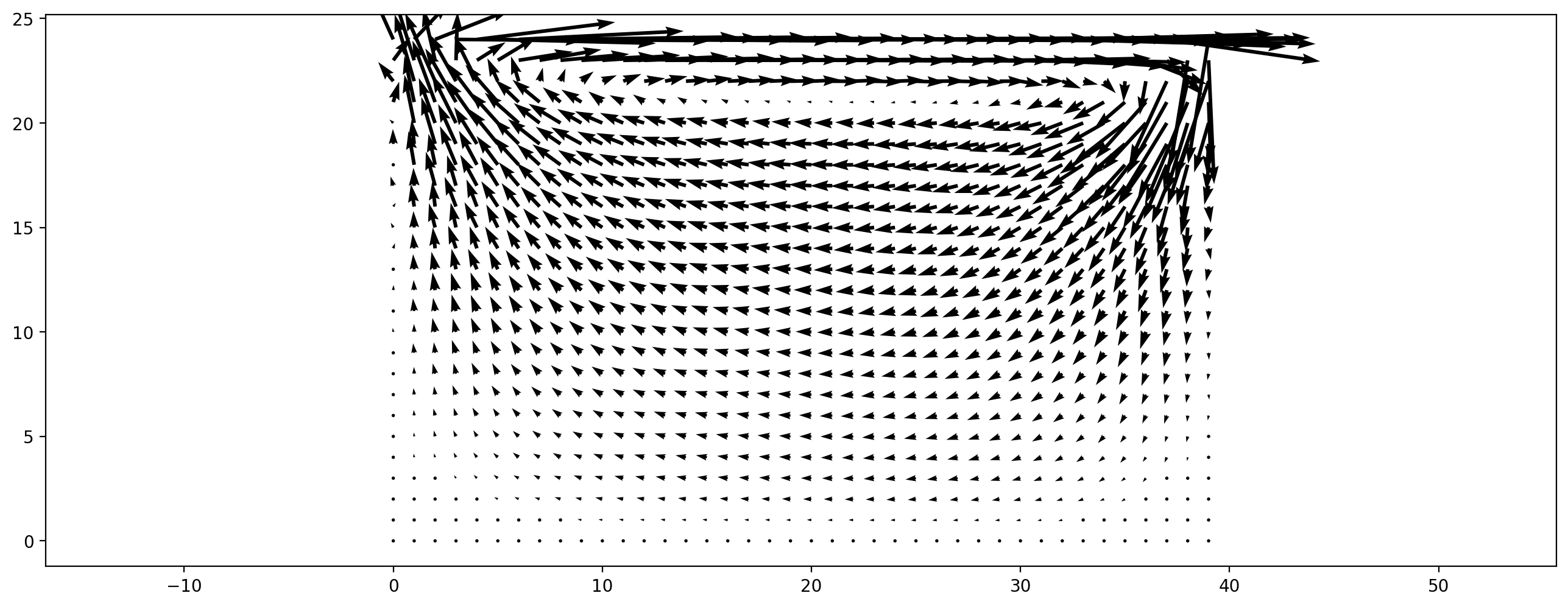In :
import pytest
pytest.importorskip('pycuda')

Out:
<module 'pycuda' from '/home/markus/miniconda3/envs/pystencils/lib/python3.8/site-packages/pycuda/__init__.py'>
In :
from lbmpy.session import *


# Tutorial 01: Running pre-defined scenarios¶

lbmpy is a module to do Lattice Boltzmann simulations in Python.

In this tutorial you will get a broad overview of lbmpy's features. We will run some of the included scenarios that come with lbmpy, like a channel flow and a lid driven cavity. This tutorial uses the simple, high-level API of lbmpy, while the following tutorials go into the low-level details.

The only prerequisite for this tutorial is basic Python and numpy knowledge.

#### What's special about lbmpy ?¶

The LBM kernels (i.e. the functions that do all the computations) are not written in Python. Instead lbmpy generates optimized C or CUDA code for these kernels and compiles it using the pystencils module. In that way we get very fast LBM kernels, a lot faster than pure Python implementations and probably also faster than handwritten C kernels. This sounds complicated, but we don't have to care about all this background work, since all compiled kernels are available as Python functions again. Thus lbmpy can be used just like any other Python package.

## Lid Driven Cavity¶

We start by simulating a fluid in a rectangular box, where one wall (the lid) is moving. This is called a 'lid driven cavity'. At the stationary walls no-slip boundary conditions are set, which enforce zero velocity at the wall. At the lid there is a velocity bounce back (UBB) boundary condition, which sets zero normal velocity and a prescribed tangential velocity.

We don't have to set up all these boundary conditions manually since there is a function create_lid_driven_cavity that does all the work for us. This function takes the tangential velocity of the lid, which drives the flow. It is given in lattice units and to get a stable simulation it should be smaller than 0.1. The relaxation_rate determines the viscosity of the fluid: Small relaxation rates correspond to high viscosity. The relaxation_rate has to be between 0 and 2.

In :
ldc_scenario = create_lid_driven_cavity(domain_size=(80,50), lid_velocity=0.01, relaxation_rate=1.95)
ldc_scenario.method

Out:
Moment Eq. Value Relaxation Rate
$1$ $\rho$ $1.95$
$x$ $u_{0}$ $1.95$
$y$ $u_{1}$ $1.95$
$x^{2}$ $\frac{\rho}{3} + u_{0}^{2}$ $1.95$
$y^{2}$ $\frac{\rho}{3} + u_{1}^{2}$ $1.95$
$x y$ $u_{0} u_{1}$ $1.95$
$x^{2} y$ $\frac{u_{1}}{3}$ $1.95$
$x y^{2}$ $\frac{u_{0}}{3}$ $1.95$
$x^{2} y^{2}$ $\frac{\rho}{9} + \frac{u_{0}^{2}}{3} + \frac{u_{1}^{2}}{3}$ $1.95$

The run method of the scenario runs the specified amount of time steps. When you run the next cell, 2000 time steps are executed and the velocity field is plotted. You can run the cell multiple times to see a time evolution.

In :
ldc_scenario.run(2000)
plt.figure(dpi=200)
plt.vector_field(ldc_scenario.velocity_slice(), step=2);### Variations to experiment with:¶

• simulate with a higher relaxation_rate (i.e. higher Reynolds number), keep in mind that the relaxation_rate has to be smaller than 2. You might have to increase (domain_size) to keep the simulation stable and run more time steps to get to the stationary solution. You also might want to increase the step parameter for the plot, to reduce the number of arrows.
• run a 3D simulation by adding a third dimension size to domain_size. The velocity property of the scenario is now a 3D field that has to be sliced before it can be plotted, e.g. ldc_scenario.velocity[:, :, 10, 0:2] generates a slice at z=10 and plot the x and y component of the velocity.

## Fully periodic flow¶

Another simple scenario is a box with periodic boundary conditions in all directions. We initialize a non-zero initial velocity field, which is decaying over time due to viscous effects and the absence of driving forces or boundary conditions. In this example we initialize a shear flow where in one stripe the fluid is moving to the left, and everywhere else to the right. We perturbe this initial velocity field with random noise to get an instable shear layer.

In :
width, height = 200, 60
velocity_magnitude = 0.05
init_vel = np.zeros((width,height,2))
# fluid moving to the right everywhere...
init_vel[:, :, 0] = velocity_magnitude
# ...except at a stripe in the middle, where it moves left
init_vel[:, height//3 : height//3*2, 0] = -velocity_magnitude
# small random y velocity component
init_vel[:, :, 1] = 0.1 * velocity_magnitude * np.random.rand(width,height)

plt.figure(dpi=200)
plt.vector_field(init_vel, step=4);